# Quick method to find line of shortest distance for skew lines

In linear algebra it is sometimes needed to find the equation of the line of shortest distance for two skew lines. What follows is a very quick method of finding that line.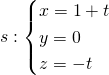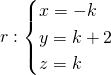(Observation: don’t make the mistake of using the same parameter for both lines. Each lines exist on its own, there’s no link between them, so there’s no reason why they should should be described by the same parameter. If this doesn’t seem convincing, get two lines you know to be intersecting, use the same parameter for both and try to find the intersection point.)

The directional vectors are:So they clearly aren’t parallel. They aren’t incidental as well, because the only possible intersection point is for, but when,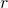is at, which doesn’t belong to. It does indeed make sense to look for the line of shortest distance between the two, confident that we will find a non-zero result.

The idea is to consider the vector linking the two lines in their generic points and then force the perpendicularity with both lines.
We will call the line of shortest distance. In our case, the vector between the generic points is (obtained as difference from the generic points of the two lines in their parametric form):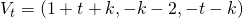Imposing perpendicularity gives us: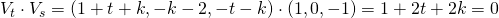Solving the two simultaneous linear equations we obtain as solution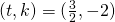.

This solution allows us to quickly get three results:

1. The equation of the line of shortest distance between the two skew lines: just replaceand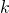in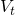with the values found. In our case,.
2. The intersection point betweenand: just replacein the parametric equation of. In our case,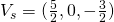.
3. The intersection point betweenand: just replacein the parametric equation of. In our case,.

Do you have a quicker method? Share it in the comments!

1.Frank Cook says:
1.Stefano Ottolenghi says: